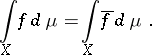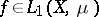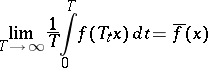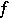# Birkhoff ergodic theorem

(diff) ← Older revision | Latest revision (diff) | Newer revision → (diff)

One of the most important theorems in ergodic theory. For an endomorphismof a spacewith a-finite measureBirkhoff's ergodic theorem states that for any functionthe limit(the time average or the average along a trajectory) exists almost everywhere (for almost all). Moreover,, and if, thenFor a measurable flowin the spacewith a-finite measureBirkhoff's ergodic theorem states that for any functionthe limitexists almost everywhere, with the same properties of.

Birkhoff's theorem was stated and proved by G.D. Birkhoff . It was then modified and generalized in various ways (there are theorems which contain, in addition to Birkhoff's theorem, also a number of statements of a somewhat different kind which are known in probability theory as ergodic theorems (cf. Ergodic theorem); there also exist ergodic theorems for more general semi-groups of transformations ). Birkhoff's ergodic theorem and its generalizations are known as individual ergodic theorems, since they deal with the existence of averages along almost each individual trajectory, as distinct from statistical ergodic theorems — the von Neumann ergodic theorem and its generalizations. (In non-Soviet literature the term "pointwise ergodic theorempointwise ergodic theorem" is often used to stress the fact that the averages are almost-everywhere convergent.)

How to Cite This Entry:
Birkhoff ergodic theorem. Encyclopedia of Mathematics. URL: http://encyclopediaofmath.org/index.php?title=Birkhoff_ergodic_theorem&oldid=12514
This article was adapted from an original article by D.V. Anosov (originator), which appeared in Encyclopedia of Mathematics - ISBN 1402006098. See original article